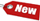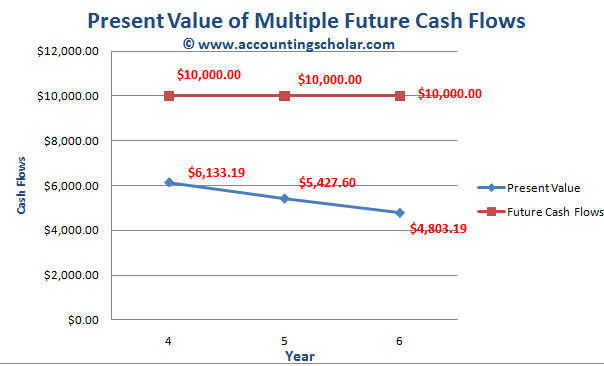Browse Accounting Lessons HereAccounting Terms & DefinitionsAccounting for Merchandising ActivitiesDebits and Credits (Double Entry Accounting)Business Valuation FormulasTime Value of Money & Present/Future ValuesComplex Debt & Equity InstrumentsCommon Stock & Shareholder's EquityAccounting & Finance RatiosValuing Common StockCorporate Income TaxesLower of Cost or Market (LCM) & Inventory ValuationChart of Accounts & BookkeepingBonds Payable & Long Term LiabilitiesCapital AssetsGAAP, Accrual & Cash Accounting, Information Commodity, Internal Controls & MaterialityWhat category of browser are you on this website? Accounting student (homework help) Finance professor (university research) Accounting manager (at work) Other Explore Careers in Accounting and FinanceVisit our section on Careers in Accounting & Finance to explore vast opportunities in this industry.

Chapter 4.14® - Calculating Present Value with Multiple Future Cash Flows – Example #2

Suppose you are offered an investment that will make three \$10,000 payments in the future (thus generating future cash flows). The first payment will occur four years from today. The second will occur five years from today and the third payment will occur in six years. If you can earn 13% on other investments, what is the most you would be willing to pay for this investment now? In other words, what is the future value of the cash flows today?

This problem is asking us to solve two problems:

 i) Future value of the cash flows in 6 years ii) Present value of the future cash flows

a) Future Value of the cash flows in 6 years

To calculate the future value of individual cash flows, here are the calculations:

Note: We will use the future value discount factor to come up with the answers.

 Future value factor = PV x (1 + r)t Year 6 = \$10,000 x 1.132 = \$12,769 Year 5 = \$10,000 x 1.131 = \$11,300 Year 4 = \$10,000 = \$10,000 Total = \$34,069

This tells us that the future value of these cash flows, at 13% rate of return is \$34,069. Notice how the funds will have grown from 3 rounds of \$10,000 contributions totalling \$30,000 to \$34,069. Thus, what is the investment gain achieved?

 Original Investments = \$10,000 x 3 = \$30,000 Future Value of Investments = \$34,069 Investment Gain = \$4,069 @ a rate of 13%

b) Present value of the future cash flows

From the value of the future cash flows in 6 years, we can easily calculate the present value using the present value discount factor below:

 Present Value Discount Factor = \$1 x [1/ (1 + r)t] = \$1/ (1 + r)t \$34,069 / 1.136 \$34,069 / 2.08 = \$16,379.33

This tell us that in order to buy this investment, the most you would be willing to pay for this investment right now (present value) is \$16,379.33 assuming a rate of return of 13%.

Alternatively, we could calculate the present value by looking at the cash flows one at a time.

 \$10,000 x 1 / 1.136 = \$4803.19 \$10,000 x 1 / 1.135 = \$5427.60 \$10,000 x 1 / 1.134 = \$6133.19 Total Present Value = \$4803.19 + \$5427.60 + \$6133.19 = \$16,363.97

Here are the results summarized in tabular and graphical format

 Year Present Value Future Cash Flows 4 \$6,133.19 \$10,000.00 5 \$5,427.60 \$10,000.00 6 \$4,803.19 \$10,000.00 Total: \$16,363.98 \$10,000.00This graph above shows the present value of the annual \$10,000 contributions each year and tells us the present value is \$6,133.19 in Year 4, \$5,427.60 in Year 5 and \$4,803.19 in Year 6. Notice as the years get older, the present value tends to get smaller.